IF YOU CAN SEE THIS go to /sfa/Nuprl/Shared/Xindentation_hack_doc.html

### Equality and Membership

Given u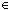A, vA, the expression "u = vA" represents the equality between u and v.

The primitive expression "u = vA" is given a meaning, whether or not it is true, only for uA and vA. See Propositions.

The reason that the type is indicated as part of the equality is that a pair of notations may be used for distinct values in one type, and yet they may stand for the same value in another type, due to the radical type-polymorphism of expressions employed in computational type theory.

For example, 2 = 4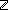mod 2, but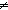4.
Or again,

Thm* (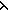x.x) = (x.if x<0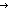0 ; x fi)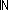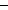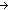,

since the first branch is never taken on, but

Thm* (x.x(x.if x<00 ; x fi)since they take different integer values on -1.

Naturally, "equality" between notations is intimately connected to notations for Functions.

Often there is a most likely type that can be discerned from context, and we elide the type from the display of an equality, though it's still in the term. For example, we may display "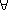f:(A). f(1) = f(2)" rather than "f:(A). f(1) = f(2)A".

Expressing Membership: tT

The expression for membership in a type is simply defined as

Def tT == t = tT.

Thus, "tT" is sensible iff it is true, and is best regarded as expressing (in t) the everywhere-true predicate on type T. In particular, it is not useful as an ordinary predicate on values of some kind, since it cannot usefully serve as an antecedent nor be negated. It is useful only when used "positively" such as in assertions of well-formedness, for example inx,y:y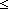x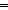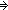x-y.

For a more thorough discussion of types, denotation and equality see Types.

(March 2001 - sfa )